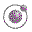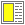The Order of Evaluation - slide 7 : 26

A motivating example - clarification

What is the value of the following expression?
`((lambda (x) 1) some-infinite-calculation)`A variation of the example in Scheme.
• Different evaluation orders give different 'results'

• The number 1

• A non-terminating calculation

• Two different semantics of function application are involved:

• Strict: A function call is well-defined if and only if all actual parameters are well-defined

• Non-strict: A function call can be well-defined even if one or more actual parameters cause an error or an infinite calculation